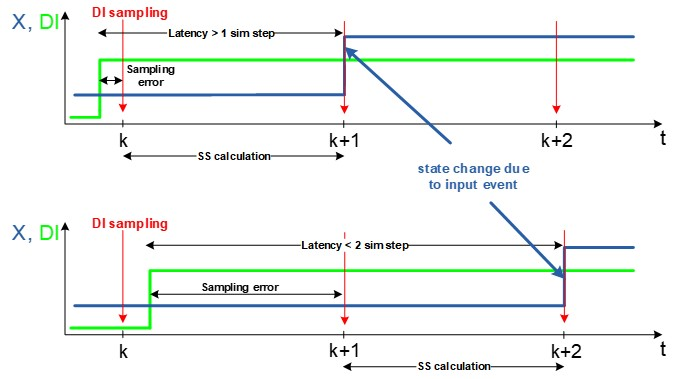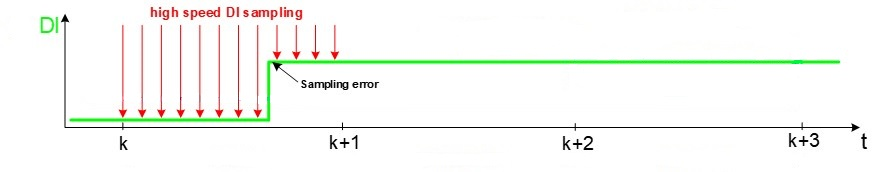# GDS oversampling

Definition and overview of GDS oversampling, and how it can be used in cases where it is needed to sample Digital inputs multiple times per simulation timestep.

Note: An interactive overview of the concepts and/or capabilities described here are available as part of the HIL Specialist 2.0 certification program on HIL Academy, as well as in the video Knowledgebase.

GDS (gate drive signals) is a digital input that is used to control the converter. In order to explain why GDS oversampling is needed and what is the the maximum frequency in which digital signals (GDSs) can be observed, let’s first define one term – the effective resolution. This resolution can be calculated by the following formula:

${\mathrm{RES}}_{\mathrm{EFF}}=⌊{\mathrm{log}}_{2}\frac{1}{{f}_{\mathrm{PWM}}{T}_{s}}⌋$ .

Since in most cases the fastest signals in a power electronics circuit are the Pulse-width modulation (PWM) gate drive signals, the effective resolution can be defined as the maximum resolution of the PWM that the HIL digital input sees for the given switching frequency. If, for example, there is a PWM signal with a resolution of 16 bits (which means 65000 different values of duty cycle), the effective resolution might be lower, depending on the PWM frequency and sampling time.

Without GDS Oversampling, GDSs are sampled with the same time steps as those defined by the simulation step. This means that if, for example, the simulation step is set to 1µS, sampling will be executed at that same moment with a sampling frequency of 1MHz. This can be a problem since, with sampling frequency this low, there is no way of knowing in which exact moment a change has occurred, which may introduce a sampling error. In this example, the effective resolution is 4-6 bits depending on the switching frequency. Figure 1 shows the effect of possible sampling errors. The green line represens the GDS which is a Digital Input (DI) and the blue line (X) represents the change in the model state after the simulation step (SS) calculation. This incorrect information about the exact time of a change in the GDS can introduce an error in the simulation results that may accumulate over time. The overall loop back latency depends when the GDS is changed with respect to the simulation steps, and it will be in the range of 1-2 simulation steps.Figure 1. DI sampling without GDS oversampling
GDS Oversampling allows the HIL device to sample GDSs multiple times in one time step. By doing this, we can attenuate sampling errors, and reach far better simulation results. With GDS Oversampling enabled, the GDS sampling period is much lower (see GDS Oversampling frequency and minimum timestep), which increases the effective resolution to around 12 or 13 bits. This means that with GDS Oversampling, we have much more accurate results. With GDS Oversampling, we can significantly increase the effective PWM resolution, as shown in Figure 2.Figure 2. GDS (DI) sampling using GDS oversampling
So far it is described how GDS oversampling is used to increase GDS sampling resolution. There are two different algorithms that rely on GDS oversampling in order to improve simulation results: Please refer to the dedicated documentation for detailed information on these two algorithms. Global GDS oversampling can be enabled from Table 2, and it is enabled by default. Switch-level GDS oversampling is implemented at the component level, and it can be enabled in the component that supports it. It is important to note that when Switch-level GDS oversampling is enabled in a component, Global GDS oversampling will be ignored for that sub-circuit, even if it is enabled in settings. However, Global GDS oversampling will be executed for all other sub-circuits (SPCs).

## GDS Oversampling frequency and minimum timestep

The GDS Oversampling resolution is defined by the IO Timing of the device. This means it can be as high as 6.25 ns for HIL402, 602+, and 604 devices, and 3.5 ns for HIL404 and HIL606 devices.